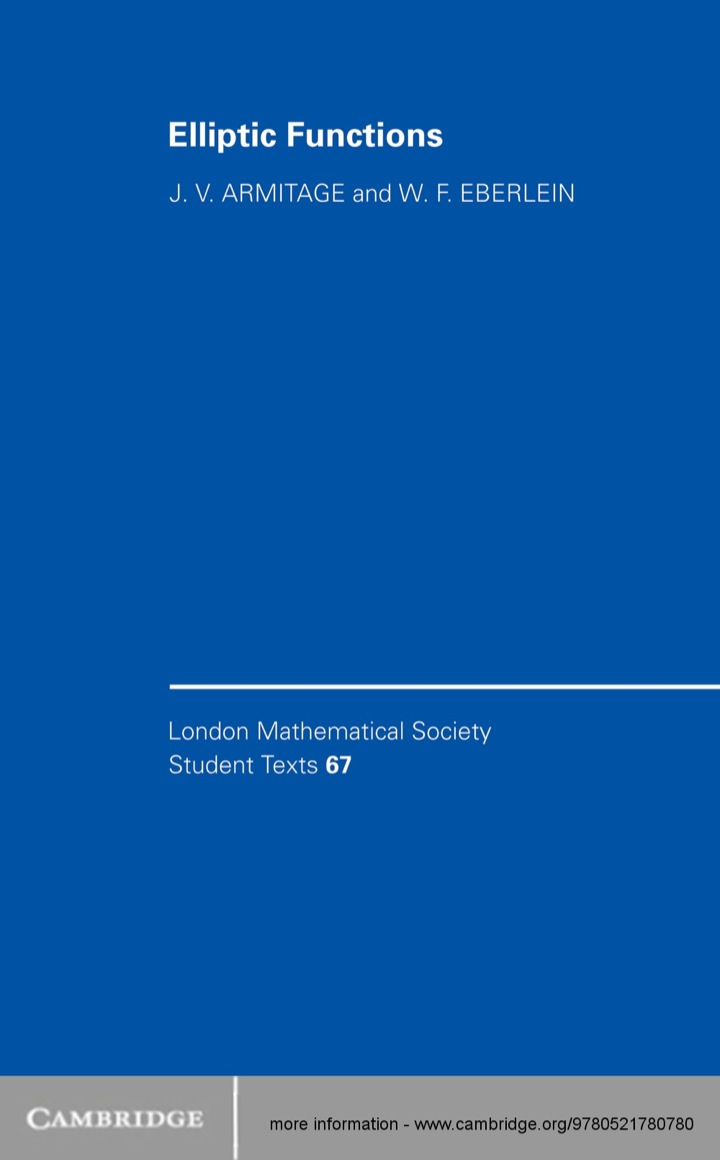# Elliptic Functions

## Elliptic Functions

By J. V. Armitage; W. F. Eberlein

### Rent or Buy eTextbook

Expires on Apr 17th, 2022
\$49

Publisher List Price: \$0.00

In its first six chapters this 2006 text seeks to present the basic ideas and properties of the Jacobi elliptic functions as an historical essay, an attempt to answer the fascinating question: 'what would the treatment of elliptic functions have been like if Abel had developed the ideas, rather than Jacobi?' Accordingly, it is based on the idea of inverting integrals which arise in the theory of differential equations and, in particular, the differential equation that describes the motion of a simple pendulum. The later chapters present a more conventional approach to the Weierstrass functions and to elliptic integrals, and then the reader is introduced to the richly varied applications of the elliptic and related functions. Applications spanning arithmetic (solution of the general quintic, the functional equation of the Riemann zeta function), dynamics (orbits, Euler's equations, Green's functions), and also probability and statistics, are discussed.

Subject: Mathematics & Statistics -> Post-Calculus -> Number Theory1st edition
Publisher: Cambridge University Press 9/28/06
Imprint: Cambridge University Press
Language: English

ISBN 10: 1107263972
ISBN 13: 9781107263970
Print ISBN: 9780521780780

Live Chats